# 1.5 Input and Output

In this section we extend the set of simple abstractions (command-line input and standard output) that we have been using as the interface between our Python programs and the outside world to include standard input, standard draw, and standard audio. Standard input makes it convenient for us to compose programs that process arbitrary amounts of input and to interact with our programs; standard draw makes it possible for us to work with graphics; and standard audio adds sound.

## Bird's-Eye View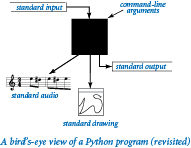The Python programs that we've seen so far take input values from the command line and write a string of characters as output. By default, both command-line input and the output written by the programs are associated with the application running on your computer that accepts commands (that is, the application in which you have been typing `python` commands). We use the generic term terminal window to refer to that application.

So far the Python programs that we've seen have used:

• Command-line input. Any Python program can access an array of strings referenced by `sys.argv`. That array contains the sequence of command-line arguments that we type, provided to Python by the operating system. By convention, both Python and the operating system process the arguments as strings, so if we intend for a command-line argument to be a number, we use a conversion function such as `int()` or `float()` to convert it from a string to the appropriate type.

• Standard output. To write output values, we have been using the functions `stdio.write()` and `stdio.writeln()`. When a program calls those functions, Python puts the results in the form of an abstract stream of characters known as standard output. By default, the operating system connects standard output to the terminal window. All of the output in our programs so far has appeared in the terminal window.

The program randomseq.py uses this model. It takes an integer command-line argument `n` and writes to standard output a sequence of `n` random numbers between 0 and 1, possibly including 0.

To complete our programming model, we add the following:

• Standard input. The booksite `stdio.py` module defines several functions in addition to `write()` and `writeln()`. Those additional functions implement a standard input abstraction to complement the standard output abstraction. That is, the `stdio` module contains functions that allow your programs to read from standard input. Just as a program can write to standard output at any time, a program can read from standard input at any time.

• Standard draw. The booksite `stddraw` module allows your programs to create and write drawings. It implements a simple graphics model that allows your programs to create and write points, lines, and geometric shapes in a window on your computer. `stddraw` also implements features for animation.

• Standard audio. The booksite `stdaudio` module allows your programs to create and play sound. It uses a standard format to convert arrays of floats into sound.

## Standard Output

This is the API of the part of the `stdio.py` module that is relevant to standard output: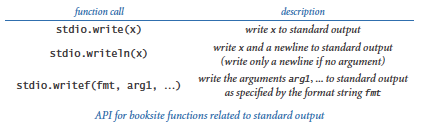The `stdio.writeln()` and `stdio.write()` functions are the ones that you have been using. The `stdio.writef()` function gives you more control over the appearance of the output, and deserves some explanation.

### Formatted writing basics.

The simplest kind of call of `stdio.writef()` passes only one argument; that argument should be a string. In that case `stdio.writef()` simply writes the string to standard output, and so is equivalent to `stdio.write()`. A more common call of `stdio.writef()` passes two arguments. In that context the first argument is called the format string. It contains a conversion specification that that describes how the second argument is to be converted to a string for output. A conversion specification has the form `%w.pc`, where: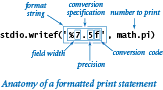• `w` is the field width. The field width is the number of characters that should be written. A negative field width indicates that the output should be padded with spaces on the right.

• `p` is the precision. For floats, the precision is the number of digits that should be written after the decimal point. For strings, the precision is the number of characters of the string that should be written.

• `c` is the conversion code. The conversion code should be `d` when writing an integer, `f` or `e` when writing a float, and `s` when writing a string.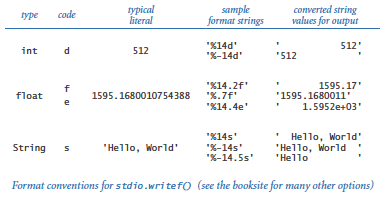The `stdio.writef()` function can take more than two arguments. In that case, the format string will have a format specifier for each argument, perhaps separated by other characters to pass through to the output.

Any part of the format string that is not a conversion specification is simply passed through to the output. For example, the statement

 ```stdio.writef('pi is approximately %.2f\n', math.pi) ```

writes the line

 ```pi is approximately 3.14 ```

### Multiple arguments.

The `stdio.writef()` function can take more than two arguments. In this case, the format string will have a conversion specification for each argument, perhaps separated by other characters to pass through to the output.

### Formatted writing implementation.

Internally the `stdio.writef()` function uses the `%` operator. Specifically, a function call of the form `stdio.writef(formatString, value)` internally is implemented using an expression of the form `formatString % value`.

As you know, the `%` operator in an expression of the form `integer % integer` means "compute the remainder". A `%` operator in an expresson of the form `formatString % value` means convert `value` to a string as directed by `formatString`. The Python documentation for string formatting operations describes the `%` operator thoroughly. Thereby, indirectly, it describes the `stdio.writef()` function thoroughly as well.

## Standard Input

Our `stdio.py` module supports standard input, an abstract data stream that may be empty or can contain a sequence of values separated by white space. Each value is a string or a value of one of Python's primitive types. One of the key features of the standard input stream is that your program consumes values when it reads them. Once your program has read a value, it cannot back up and read it again. This is the part of the `stdio` module that is relevant to standard input: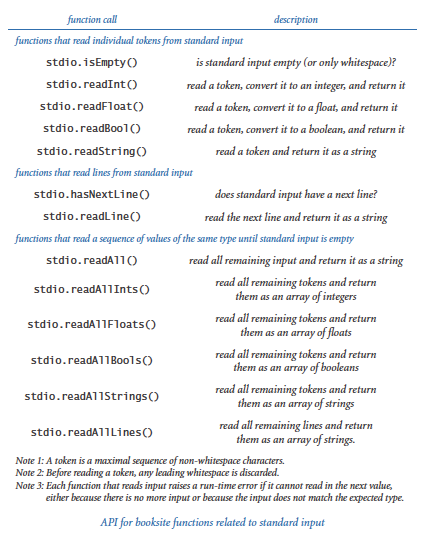These functions fall into one of three categories: those for reading individual tokens, one at a time, and converting each to an integer, float, boolean or string; those for reading lines from standard input, one at a time; and those for reading a sequence of values of the same type (returning the values in an array). Generally, it is best not to mix functions from the different categories in the same program.

We now consider a few example programs that illustrate how to use standard input.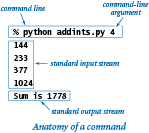### Typing input.

The program addints.py accepts a command-line argument `n`. Then it reads `n` numbers from standard input, computes their sum, and writes the sum to standard output.

When you use the `python` command to run a Python program from the command line, you actually are doing three things: (i) issuing a command to start executing your program, (ii) specifying the values of the command-line arguments, and (iii) beginning to define the standard input stream. The string of characters that you type in the terminal window after the command line is the standard input stream. When you type characters, you are interacting with your program. The program waits for you to create the standard input stream.

When you type `python addints.py`, after accepting the command-line argument the program calls `stdio.readInt()` and waits for you to type an integer. Suppose that you want `144` to be the first input: as you type `1`, then `4`, and then `4`, nothing happens, because `stdio` does not know that you are done typing the integer, but when you then type `<return>` to signify the end of your integer, `stdio.readInt()` immediately returns the value 144. After you have typed four numbers in this way, the program expects no more input and writes the sum to standard output, as desired.

If you type `abc` or `12.2` or `True` when `stdio.readInt()` is expecting an integer, then it will respond with a `ValueError`. The format for each type is the same as you have been using for literal values within Python programs. `stdio` treats strings of consecutive whitespace characters as identical to one space and allows you to delimit your numbers with such strings. It doesn't matter how many spaces you put between numbers, whether you enter numbers on one line or separate them with tab characters or spread them out over several lines, (except that your terminal application processes standard input one line at a time, so it will wait until you type `<return>` before sending all of the numbers on that line to standard input). You can mix values of different types in an input stream, but each time that the program expects a value of a particular type, there needs to be a value of that type in the input stream.

### Interactive user input.

The program twentyquestions.py plays a simple guessing game. You type numbers, each of which is an implicit question (Is this the number?) and the program tells you whether your guess is too high or too low.

That program illustrates interactive user input. That is, it illustrates that a program can interleave writing to standard output and reading from standard input, thereby interacting with the user during program execution.

### Processing an arbitrary-size input stream

The program average.py reads a sequence of floats from standard input and writes their average to standard output.

Typically, input streams are finite: your program marches through the input stream, consuming values until the stream is empty. But there is no restriction on the size of the input stream. That program illustrates a key property of using an input stream: the length of the stream is not known to the program. We type all the numbers that we have, then the program averages them. Before reading each number, the program calls `stdio.isEmpty()` to check whether there are any more numbers in standard input.

How do you signal that we have no more data to type? By convention, we type a special sequence of characters known as the end-of-file sequence. It is `<ctrl-d>` on OS X and Linux, and it is `<ctrl-z>` on Windows. On some systems the end-of-file sequence must appear on its own line. Typing the end-of-file sequence indicates that standard input is empty.

## Redirection and Piping

For many applications, typing input data as a standard input stream from the terminal window is untenable because doing so limits our program's processing power by the amount of data that we can type. Similarly, we often want to save the information printed on the standard output stream for later use. We can use operating system mechanisms to address both issues.

### Redirecting standard output to a file.

By adding a simple directive to the command that invokes a program, we can redirect its standard output to a file, for permanent storage or for input to some other program at a later time. For example, the command: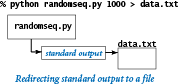specifies that the standard output stream is not to be written in the terminal window, but instead is to be written to a text file named `data.txt`. Each call to `stdio.write()` or `stdio.writeln()` appends text at the end of that file. In this example, the end result is a file that contains 1,000 random values. No output appears in the terminal window: it goes directly into the file named after the `>` symbol. Thus, we can save away information for later retrieval.

### Redirecting standard input from a file.

Similarly, we can redirect standard input so that a program reads data from a file instead of the terminal application. For example, the command: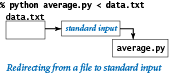reads a sequence of numbers from the file `data.txt`, computes their average, and writes the average to standard output. Specifically, the `<` symbol is a directive to implement the standard input stream by reading from the file `data.txt` instead of by waiting for the user to type something into the terminal window. When the program calls `stdio.readFloat()`, the operating system reads the value from the file. This facility to redirect standard input from a file enables us to process huge amounts of data from any source with our programs, limited only by the size of the files that we can store.

### Connecting two programs.

The most flexible way to implement the standard input and standard output abstractions is to specify that they are implemented by our own programs! This mechanism is called piping. For example, the following command: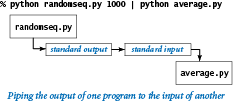specifies that the standard output stream for randomseq.py and the standard input stream for average.py are the same stream. That is, the result has the same effect as the following sequence of commands:

```% python randomseq.py 1000 > data.txt
% python average.py < data.txt
```

but the file `data.txt` is not needed.

### Filters.

For many common tasks, it is convenient to think of each program as a filter that converts a standard input stream to a standard output stream in some way, with piping as the command mechanism to connect programs together. For example, rangefilter.py takes two command-line arguments and writes to standard output those numbers from standard input that fall within the specified range.

Several standard filters that were designed for Unix still survive (sometimes with different names) as commands in modern operating systems. For example, the `sort` filter reads the lines from standard input and writes them to standard output in sorted order:

 ```% python randomseq.py 9 | sort 0.0472650078535 0.0681950168757 0.0967410236589 0.0974385525393 0.118855769243 0.46604926859 0.522853708616 0.599692836211 0.685576779833 ```

Another useful filter is `more`, which reads data from standard input and displays it in your terminal window one screenful at a time. For example, if you type

 ```% python randomseq.py 1000 | more ```

you will see as many numbers as fit in your terminal window, but more will wait for you to hit the space bar before displaying each succeeding screenful.

## Standard Drawing

Now we introduce an abstraction for producing drawings as output. We imagine an abstract drawing device capable of drawing lines and points on a two-dimensional "canvas" and then displaying that canvas on your screen in the standard drawing window. The device is capable of responding to the commands that our programs issue in the form of calls to functions in the `stddraw` module. The module's API consists of two kinds of functions: drawing functions that cause the device to take an action (such as drawing a line or drawing a point) and control functions that control how the drawing is shown and set parameters such as the pen size or the coordinate scales.

### Creating drawings.

The basic functions for drawing are described in this API: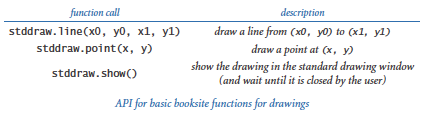The drawing functions are nearly self-documenting: `stddraw.line()` draws a straight line segment connecting two points whose coordinates are given as arguments and `stddraw.point()` draws a dot centered at the given coordinates. The default coordinate scale is the unit square (all coordinates between 0 and 1). The point (0.0, 0.0) is at the lower left, and the point (1.0, 1.0) is at the upper right — thus corresponding to the first quadrant of the familiar Cartesian coordinate system. The default settings draw black lines and black points on a white background.

The control function stddraw.show() needs a bit more explanation. When your program calls any drawing function such as `stddraw.line()` or `stddraw.point()`, `stddraw` uses an abstraction known as the background canvas. The background canvas is not displayed; it exists only in computer memory. All points, lines, and so forth are drawn on the background canvas, not directly in the standard drawing window. Only when you call `stddraw.show()` does your drawing get copied from the background canvas to the standard drawing window, where it is displayed until the user closes the standard drawing window — typically by clicking on the Close button in the window's title bar.

Why does `stddraw` need to use a background canvas? The main reason is that use of two canvases instead of one makes the `stddraw` module more efficient. Incrementally displaying a complex drawing as it is being created can be intolerably inefficient on many computer systems. In computer graphics, this technique is known as double buffering.

To summarize the information that you need to know, a typical program using the stddraw module has this structure:

• Import the `stddraw` module.
• Call drawing functions such as `stddraw.line()` and `stddraw.point()` to create a drawing on the background canvas.
• Call `stddraw.show()` to show the background canvas in the standard drawing window and wait until the window is closed.

### Your first drawing.

The "Hello, World" equivalent for graphics programming with `stddraw` is to draw a triangle with a point inside. To form the triangle, we draw three lines. The program triangle.py is the full program.

### Saving a drawing.

You can save the standard drawing window canvas to a file. To do so, right-click anywhere on the window canvas. After you do that, `stddraw` displays a file dialog box which allows you to specify a file name. Then, after you type a file name into the dialog box and click the Save button, `stddraw` saves the window canvas to a file with the specified name. The file name must end with either `.jpg` (to save the window canvas in JPEG format) or `.png` (to save the window canvas in "Portable Network Graphics" format). The drawings generated by the graphics programs shown in this chapter were saved to files using this mechanism.

### Control commands.

The default coordinate system for standard drawing is the unit square, but we often want to draw plots at different scales. Also, we often want to draw lines of different thickness and points of different size from the standard. To accommodate these needs, stddraw has these functions: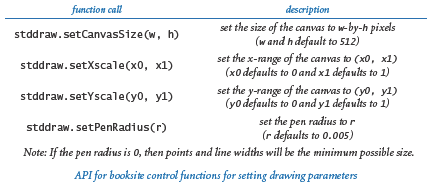For example, when you call the function `stddraw.setXscale(0, n)`, you are telling the drawing device that you will be using x-coordinates between 0 and `n`. Note that the two-call sequence

 ```stddraw.setXscale(x0, x1) stddraw.setYscale(y0, y1) ```

sets the drawing coordinates to be within a bounding box whose lower-left corner is at (`x0`, `y0`) and whose upper-right corner is at (`x1`, `y1`).

### Filtering data to a standard drawing.

The program plotfilter.py reads a sequence of points defined by (x, y) coordinates and draws a spot at each point. It adopts the convention that the first four numbers read from standard input specify the bounding box, so that it can scale the plot. Try running it with its standard input redirected to usa.txt.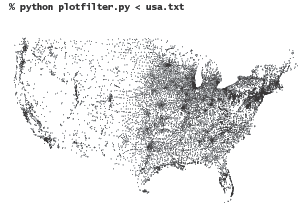### Plotting a function graph.

The program functiongraph.py plots the function y = sin(4x) + sin(20x) in the interval (0, π). There are an infinite number of points in the interval, so we have to make do with evaluating the function at a finite number of points within the interval. We sample the function by choosing a set of x values, then computing y values by evaluating the function at each x value. Plotting the function by connecting successive points with lines produces what is known as a piece-wise linear approximation.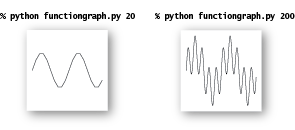### Outline and filled shapes.

The `stddraw` module also includes functions to draw circles, rectangles, and arbitrary polygons. Each shape defines an outline. When the function name is just the shape name, that outline is traced by the drawing pen. When the name begins with `filled`, the named shape is instead filled solid, not traced. As usual, we summarize the available functions in an API: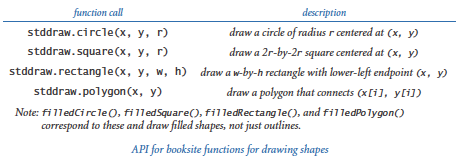The arguments for `stddraw.circle()` define a circle of radius `r` centered at (`x`, `y`); the arguments for `stddraw.square()` define a square of side length 2`r` centered at (`x`, `y`); and the arguments for `stddraw.polygon()` define a sequence of points that we connect by lines, including one from the last point to the first point.

### Text and color.

To annotate or highlight various elements in your drawings, `stddraw` includes methods for drawing text, setting the font, and setting the the ink in the pen.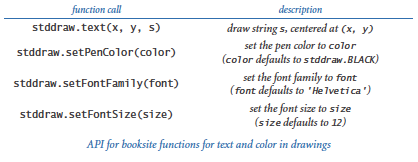In this code, color and fonts use types that you will learn about in Section 3.1. Until then, we leave the details to `stddraw`. The available pen colors are `BLACK`, `BLUE`, `CYAN`, `DARK_GRAY`, `GRAY`, `GREEN`, `LIGHT_GRAY`, `MAGENTA`, `ORANGE`, `PINK`, `RED`, `WHITE`, and `YELLOW`, defined as constants within `stddraw`. For example, the call `stddraw.setPenColor(stddraw.GRAY)` changes to gray ink. The default ink color is black; the default font is a 12-point plain Helvetica font.

These code fragments illustrate some stddraw functions for drawing shapes and text: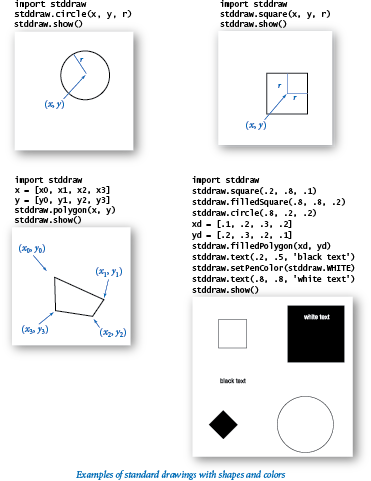### Animation

If we provide an argument to `stddraw.show()`, then that call need not be the last action of a program: it will copy the background canvas to the standard drawing window and then wait for the specified number of milliseconds. As you will soon see, we can use this capability (coupled with the ability to erase, or clear the background canvas) to produce the effect of motion in the `stddraw` window.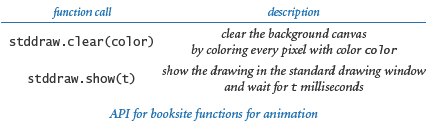The "Hello, World" program for animation is to produce a black ball that appears to move around on the canvas. Suppose that the ball is at position (rx , ry) and we want to create the impression of moving it to a new position nearby, such as, for example, (rx + 0.01, ry + 0.02). We do so in three steps:

• Clear the background canvas.
• Draw a black ball at the new position.
• Show the drawing and wait for a short while.

To create the illusion of movement, we iterate these steps for a whole sequence of positions (one that will form a straight line, in this case). The argument to `stddraw.show()` quantifies "a short while" and controls the apparent speed.

The program bouncingball.py implements these steps to create the illusion of a ball moving in the 2-by-2 box centered at the origin. The current position of the ball is (rx , ry), and we compute the new position at each step by adding vx to rx and vy to ry. Since (vx , vy) is the fixed distance that the ball moves in each time unit, it represents the velocity. To keep the ball in the drawing, we simulate the effect of the ball bouncing off the walls according to the laws of elastic collision. This effect is easy to implement: when the ball hits a vertical wall, we just change the velocity in the x-direction from vx to -vx, and when the ball hits a horizontal wall, we change the velocity in the y-direction from vy to -vy. The images below, which show the track of the ball, are produced by a modified version of this code (see an exercise at the end of this section).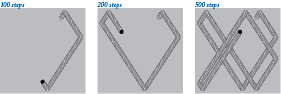## Standard Audio

The stdaudio module can play, manipulate, and synthesize sound. It allows you to play `.wav` files, to compose programs to create and manipulate arrays of floats, and to read and write them as .wav files: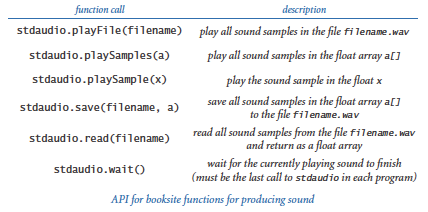We first introduce some some basic concepts behind one of the oldest and most important areas of computer science and scientific computing, which is known as digital signal processing.

### Concert A.

Sound is the perception of the vibration of molecules — in particular, the vibration of our eardrums. Therefore, oscillation is the key to understanding sound. Perhaps the simplest place to start is to consider the musical note A above middle C, which is known as concert A. This note is nothing more than a sine wave, scaled to oscillate at a frequency of 440 times per second. The function sin(t) repeats itself once every 2π units, so if we measure t in seconds and plot the function sin(2πt × 440), we get a curve that oscillates 440 times per second. We measure frequency in hertz (cycles per second). When you double or halve the frequency, you move up or down one octave on the scale. For example, 880 hertz is one octave above concert A and 110 hertz is two octaves below concert A. For reference, the frequency range of human hearing is about 20 to 20,000 hertz. The amplitude (y-value) of a sound corresponds to the volume. We assume it is scaled to be betwen -1 and +1.

### Other notes.

A simple mathematical formula characterizes the other notes on the chromatic scale. There are 12 notes on the chromatic scale, divided equally on a logarithmic (base 2) scale. We get the ith note above a given note by multiplying its frequency by the (i/12)th power of 2. In other words, the frequency of each note in the chromatic scale is precisely the frequency of the previous note in the scale multiplied by the twelfth root of 2 (about 1.06). This information suffices to create music! For example, to play the tune Frere Jacques, we just need to play each of the notes A B C# A by producing sine waves of the appropriate frequency for about half a second and then repeat the pattern.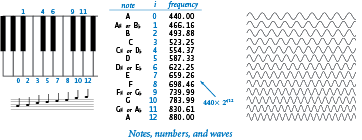### Sampling.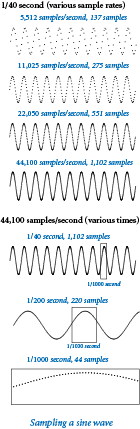For digital sound, we represent a curve by sampling it at regular intervals, in precisely the same manner as when we plot function graphs. We sample sufficiently often that we have an accurate representation of the curve &mdot; a widely used sampling rate for digital sound is 44,100 samples per second. For concert A, that rate corresponds to plotting each cycle of the sine wave by sampling it at about 100 points. Since we sample at regular intervals, we need to compute only the y coordinates of the sample points. It is that simple: we represent sound as an array of numbers (float values that are between -1 and +1). Our booksite sound module function `stdaudio.playSamples()` takes an array of floats as its argument and plays the sound represented by that array on your computer.

For example, suppose that you want to play concert A for 10 seconds. At 44,100 samples per second, you need an array of 441,001 float values. To fill in the array, use a `for` loop that samples the function sin(2πt × 440) at t = 0/44100, 1/44100, 2/44100, 3/44100, ..., 441000 / 44100. Once we fill the array with these values, we are ready for `stdaudio.playSamples()`, as in the following code:

 ```import math import stdaudio import stdarray SPS = 44100 # samples per second hz = 440.0 # concert A duration = 10.0 # ten seconds n = int(SPS * duration) a = stdarray.create1D(n+1) for i in range(n+1): a[i] = math.sin(2.0 * math.pi * i * hz / SPS) stdaudio.playSamples(a) stdaudio.wait() ```

This code is the "Hello, World" of digital audio. Once you use it to get your computer to play this note, you can compose code to play other notes and make music!

### Saving to a file.

Music can take up a lot of space on your computer. At 44,100 samples per second, a four-minute song corresponds to 4 × 60 × 44100 = 10,584,000 numbers. Therefore, it is common to represent the numbers corresponding to a song in a binary format that uses less space than the string-of-digits representation that we use for standard input and output. Many such formats have been developed in recent years — `stdaudio` uses the `.wav` format.

### Playing tunes.

The program playthattune.py is an example that shows how easily we can create music with `stdaudio`. It takes notes from standard input, indexed on the chromatic scale from concert A, and plays them on standard audio. Try running it repeatedly with its standard input redirected to each of these data files (created by various students): elise.txt, ascale.txt, stairwaytoheaven.txt, entertainer.txt, firstcut.txt, freebird.txt, and looney.txt.

#### Q & A

Q. How can I make the booksite modules stdio, stddraw, and stdaudio available to Python?

A. If you followed the step-by-step instructions on this booksite for installing Python, these modules should already be available to Python.

Q. Are there standard Python modules for handling standard output?

A. Actually, such features are built into Python. In Python 2, you can use the `print` statement to write data to standard output. In Python 3, there is no `print` statement; instead, there is a `print()` function, which is similar.

Q. Why, then, are we using the booksite stdio module for writing to standard output instead of using the features already provided by Python?

A. Our intention is to compose code that works (as much as possible) with all versions of Python. For example, using the `print` statement in all our programs would mean they would work with Python 2, but not with Python 3. Since we use `stdio` functions, we just need to make sure that we have the proper library.

Q. How about standard input?

A. There are (different) capabilities in Python 2 and Python 3 that correspond to `stdio.readLine()`, but nothing corresponding to `stdio.readInt()` and similar functions. Again, by using `stdio`, we can compose programs that not just take advantage of these additional capabilities, but also work in both versions of Python.

Q. How about drawing and sound?

A. Python does not come with an `audio` library. Python comes with a graphics library named `Tkinter` for producing drawings, but it is too slow for some of the graphics applications in the book. Our `stddraw` and `stdaudio` modules provide easy-to-use APIs, based on the `Pygame` library.

Q. So, let me get this straight; if I use the format `%2.4f` with `stdio.writef()` to write a float, I get two digits before the decimal point and four digits after the decimal point, right?

A. No, that specifies just four digits after the decimal point. The number preceding the decimal point is the width of the whole field. You want to use the format `%7.2f` to specify seven characters in total — four before the decimal point, the decimal point itself, and two digits after the decimal point.

Q. Which other conversion codes are there for `stdio.writef()`?

A. For integer values, there are `o` for octal, `x` for hexadecimal; for floating point, you can use `e` or `g` to get scientific notation. There are also numerous formats for dates and times. The Python documentation for string formatting operations provides a wealth of information.

Q. Can my program reread data from standard input?

A. No. You get only one shot at it, in the same way that you cannot undo a call of `stdio.writeln()`.

Q. What happens if my program attempts to read data from standard input after it is exhausted?

A. Python will raise an `EOFError` at run time. The functions `stdio.isEmpty()` and `stdio.hasNextLine()` allow you to avoid such an error by checking whether more input is available.

Q. Why does `stddraw.square(x, y, r)` draw a square of width 2`r` instead of `r`?

A. This makes it consistent with the function `stddraw.circle(x, y, r)`, where the third argument is the radius of the circle, not the diameter. In this context, `r` is the radius of the biggest circle that can fit inside the square.

Q. What happens if my program calls `stddraw.show(0)`?

A. That function call tells stddraw to copy the background canvas to the standard drawing window, and then wait 0 milliseconds (that is, do not wait at all) before proceeding. That function call is appropriate if, for example, you want to run an animation at the fastest rate supported by your computer.

Q. Can I draw curves other than circles with `stddraw`?

A. We had to draw the line somewhere (pun intended), so we support only the basic shapes discussed in the text. You can draw other shapes one point at a time, as explored in several exercises in the text, but filling them is not directly supported.

Q. So I use negative integers to go below concert A when making input files for playthattune.py?

A. Right. Actually, our choice to put concert A at 0 is arbitrary. A popular standard, known as the MIDI Tuning Standard, starts numbering at the C five octaves below concert A. By that convention, concert A is 69 and you do not need to use negative numbers.

Q. Why do I hear weird results from standard audio when I try to sonify a sine wave with a frequency of 30,000 hertz (or more)?

A. The Nyquist frequency, defined as one-half the sampling frequency, represents the highest frequency that can be reproduced. For standard audio, the sampling frequency is 44,100, so the Nyquist frequency is 22,050.

Q. How do I enter the end-of-file sequence if I am redirecting standard input from a file?

A. When standard input is bound to your terminal application, you eventually must enter the end-of-file sequence to inform the program that no more data remains to be read from standard input. However when standard input is bound to a file, you need not enter the end-of-file sequence. Instead the operating system automatically informs your program when no more data remains to be read from the file.

Q. What other conversion codes are there for `stdio.writef()`?

Q. How do I print the `%` character within `stdio.writef()`?

A. Use `%%`.

Q. What is the symbol for the end of a line?

A. Different operating systems use different symbols. On Unix systems and Mac OS X, the newline character is `'\n'`. On Windows each line is terminated by a string of two characters `'\r\n'`. On Macs prior to OS X, each line is terminated by the string `'\n\r'`. When composing a program, you should avoid using operating system specific features or else it might not work as expected on other systems. Use `stdio.writeln()` to write a newline.

Q. How do I create colors for use with the the `stddraw` module?

A. The stddraw module uses a class named `Color` that we defined specifically for this booksite. Chapter 3 of the booksite describes that class.

Q. What are the main differences of the PNG, JPEG, and PostScript graphics formats?

A. The graphics on most web pages are in PNG, GIF, or JPEG format. All three formats are raster-based — they store the set of pixels and color gradations needed to represent a picture. PNG and GIF are ideal for displaying figures with straight lines and geometric figures, while JPEG is best suited for photographs. PostScript is a vector-based format. For example, it represents a circle as a geometric object instead of a collection of thousands of pixels. The quality does not degrade if you enlarge or shrink it. For this reason, most printers use PostScript to print documents and graphics.

Q. What does the error message `NameError: name 'stdio' is not defined` mean?

A. You probably forgot to install the booksite modules. Of course the same applies to `stdarray.py`, `stddraw.py`, and `stdaudio.py`.

Q. How can I create an animated GIF?

#### Exercises

1. Compose a program that reads in integers (as many as the user enters) from standard input and writes the maximum and minimum values to standard output.

Solution: See maxmin.py.

2. Modify your program from the previous exercise to insist that the integers must be positive (by prompting the user to enter positive integers whenever the value entered is not positive).

3. Compose a program that accepts an integer n from the command line, reads n floats from standard input, and writes their mean (average value) and standard deviation (square root of the sum of the squares of their differences from the average, divided by n).

Solution: See stats2.py.

4. Extend your program from the previous exercise to create a filter that writes all the values that are further than 1.5 standard deviations from the mean.

5. Compose a program that reads in a sequence of integers and writes both the integer that appears in a longest consecutive run and the length of the run. For example, if the input is `1 2 2 1 5 1 1 7 7 7 7 1 1`, then your program should write `Longest run: 4 consecutive 7s`.

Solution: See longestrun.py.

6. Compose a filter that reads in a sequence of integers and writes the integers, removing repeated values that appear consecutively. For example, if the input is `1 2 2 1 5 1 1 7 7 7 7 1 1 1 1 1 1 1 1 1`, your program should write `1 2 1 5 1 7 1`.

7. Compose a program that accepts a command-line argument `n`, reads from standard input `n-1` distinct integers between 1 and `n`, and determines the missing value.

8. Compose a program that reads in positive real numbers from standard input and writes their geometric and harmonic means. The geometric mean of n positive numbers x1, x2, ..., xn is (x1 × x2 × ... × xn)1/n. The harmonic mean is n / (1/x1 + 1/x2 + ... + 1/xn). Hint: For the geometric mean, consider taking logarithms to avoid overflow.

9. Suppose that the file `input.txt` contains the two strings F and F. What does the following command do? See the exercises from Section 1.2 for more information on dragon curves. Here is the Python program dragon3.py.

 ```python dragon3.py < input.txt | python dragon3.py | python dragon3.py ```
10. Compose a filter `tenperline.py` that reads a sequence of integers between 0 and 99 and writes 10 integers per line, with columns aligned. Then compose a program `randomintseq.py` that takes two command-line arguments `m` and `n` and writes `n` random integers between 0 and `m`-1. Test your programs with the command `python randomintseq 100 200 | python tenperline.py`.

11. Compose a program named `wordcount.py` that reads text from standard input and writes to standard output the number of words in the text. For the purpose of this exercise, a word is a sequence of non-whitespace characters that is surrounded by whitespace. For example, the command `python wordcount < tale.txt` should write 139043.

Solution: See wordcount.py.

12. Compose a program that reads lines from standard input with each line containing a name and two integers, and then calls `stdio.writef()` to write to standard output a table with a column of the names, the integers, and the result of dividing the first by the second, accurate to three decimal places. You could use a program like this to tabulate batting averages for baseball players or grades for students.

13. Which of the following require saving all the values from standard input (in an array, say), and which could be implemented as a filter using only a fixed number of variables? For each, the input comes from standard input and consists of n floats between 0 and 1.

• Write the maximum and minimum numbers.
• Write the kth smallest value.
• Write the sum of the squares of the numbers.
• Write the average of the numbers.
• Write the percentage of numbers greater than the average.
• Write the numbers in increasing order.
• Write the numbers in random order.
14. Compose a program that writes a table of the monthly payments, remaining principal, and interest paid for a loan, taking three numbers as command-line arguments: the number of years, the principal, and the interest rate. (See a related exercise in Section 1.2.)

15. Compose a program that takes three command-line arguments x, y, and z, reads from standard input a sequence of point coordinates (xi, yi, zi), and writes the coordinates of the point closest to (x, y, z). Recall that the square of the distance between (x , y , z) and (xi , yi , zi ) is (x - xi)2 + (y - yi)2 + (z - zi)2. For efficiency, do not use either `math.sqrt()` or the `**` operator.

Solution: See closest.py.

16. Compose a program that, given the positions and masses of a sequence of objects, computes their center-of-mass, or centroid. The centroid is the average position of the n objects, weighted by mass. If the positions and masses are given by (xi, yi, mi), then the centroid (x, y, m) is given by

m = m1 + m2 + ... + mn x = (m1x1 + ... + mnxn) / m y = (m1y1 + ... + mnyn) / m
17. Compose a program that reads in a sequence of floats between -1 and 1 and writes their average magnitude, average power, and the number of zero crossings. The average magnitude is the average of the absolute values of the data values. The average power is the average of the squares of the data values. The number of zero crossings is the number of times a data value transitions from a strictly negative number to a strictly positive number, or vice versa. These three statistics are widely used to analyze digital signals.

18. Compose a program that takes an integer command-line argument `n` and plots an `n`-by-`n` checkerboard with red and black squares. Color the lower left square red.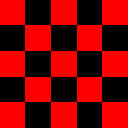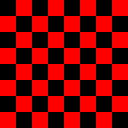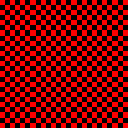Solution: See checkerboard.py

19. Compose a program that takes as command-line arguments an integer `n` and a float `p` (between 0 and 1), plots `n` equally spaced points of size on the circumference of a circle, and then, with probability `p` for each pair of points, draws a gray line connecting them.20. Compose code to draw hearts, spades, clubs, and diamonds. To draw a heart, draw a diamond, then attach two semicircles to the upper left and upper right sides.

21. Compose a program that takes an integer command-line argument `n` and plots a "flower" with `n` petals (if `n` is odd) or `2n` petals (if `n` is even) by plotting the polar coordinates (r, θ) of the function r = sin(n × θ) for θ ranging from 0 to `2π` radians. Below is the desired output for `n` = 4, 7, and 8.22. Solution: See rose.py.

23. Compose a program that takes a string `s` from the command line and displays it in banner style on the screen, moving from left to right and wrapping back to the beginning of the string as the end is reached. Add a second command-line argument to control the speed.

Solution: See banner.py.

24. Modify playthattune.py to take additional command-line arguments that control the volume (multiply each sample value by the volume) and the tempo (multiply each note's duration by the tempo).

25. Compose a program that takes the name of a `.wav` file and a playback rate r as command-line arguments and plays the file at the given rate. First, use `stdaudio.read()` to read the file into an array `a[]`. If r = 1, just play `a[]`; otherwise create a new array `b[]` of approximate size r times `len(a)`. If r < 1, populate `b[]` by sampling from the original; if r > 1, populate `b[]` by interpolating from the original. Then play `b[]`.

26. Compose programs that use `stddraw` to create each of these designs.27. Compose a program that draws filled circles of random size at random positions in the unit square, producing images like those below. Your program should take four command-line arguments: the number of circles, the probability that each circle is black, the minimum radius, and the maximum radius.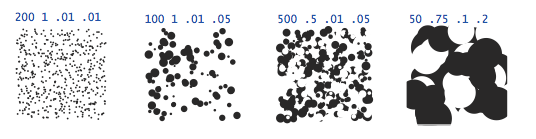#### Creative Exercises

1. Visualizing audio. Modify playthattune.py to send the values played to standard drawing, so that you can watch the sound waves as they are played. You will have to experiment with plotting multiple curves in the drawing canvas to synchronize the sound and the picture.

2. Statistical polling. When collecting statistical data for certain political polls, it is very important to obtain an unbiased sample of registered voters. Assume that you have a file with n registered voters, one per line. Compose a filter that writes a random sample of size m. (See the sample.py program from Section 1.4.)

3. Terrain analysis. Suppose that a terrain is represented by a two-dimensional grid of elevation values (in meters). A peak is a grid point whose four neighboring cells (left, right, up, and down) have strictly lower elevation values. Compose a program that reads a terrain from standard input and then computes and writes the number of peaks in the terrain.

4. Histogram. Suppose that the standard input stream is a sequence of floats. Compose a program that takes an integer `n` and two floats `lo` and `hi` from the command line and uses `stddraw` to plot a histogram of the count of the numbers in the standard input stream that fall in each of the `n` intervals defined by dividing (`lo`, `hi`) into `n` equal-sized intervals.

5. Spirographs. Compose a program that accepts three command-line arguments R, r, and a and draws the resulting spirograph. A spirograph (technically, an epicycloid) is a curve formed by rolling a circle of radius r around a larger fixed circle or radius R. If the pen offset from the center of the rolling circle is (r+a), then the equation of the resulting curve at time t is given by

 ```x(t) = (R+r)cos(t) - (r+a)cos((R+r)t/r) y(t) = (R+r)sin(t) - (r+a)sin((R+r)t/r) ```

Such curves were popularized by a best-selling toy that contains discs with gear teeth on the edges and small holes that you could put a pen in to trace spirographs.

Solution: See spirograph.py.

6. Clock. Compose a program that displays an animation of the second, minute, and hour hands of an analog clock. Use the call `stddraw.show(1000)` to update the display roughly once per second.

Hint: this may be one of the rare times when you want to use the `%` operator with a float; it works the way you would expect.

Solution: See clock.py.

7. Oscilloscope. Compose a program to simulate the output of an oscilloscope and produce Lissajous patterns. These patterns are named after the French physicist, Jules A. Lissajous, who studied the patterns that arise when two mutually perpendicular periodic disturbances occur simultaneously. Assume that the inputs are sinusoidal, so that the following parametric equations describe the curve:

 ```x(t) = Ax sin (wxt + θx) y(t) = Ay sin (wyt + θy) ```

Take the six parameters Ax and Ay (amplitudes); wx and wy (angular velocity); and θx and θy (phase factors) from the command line.

For example, the first image below has Ax = Ay = 1, wx = 2, wy = 3, θx = 20 degrees, θy = 45 degrees. The other has parameters (1, 1, 5, 3, 30, 45)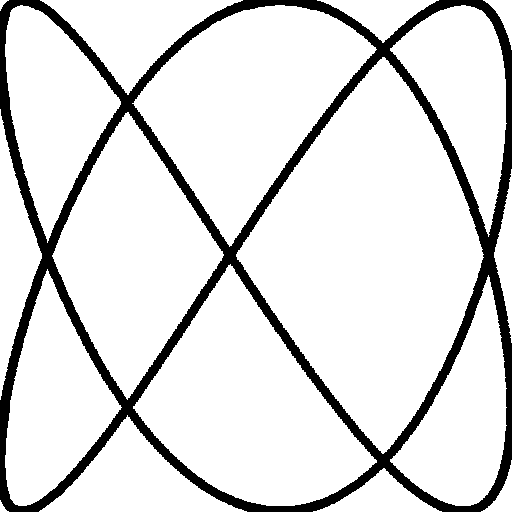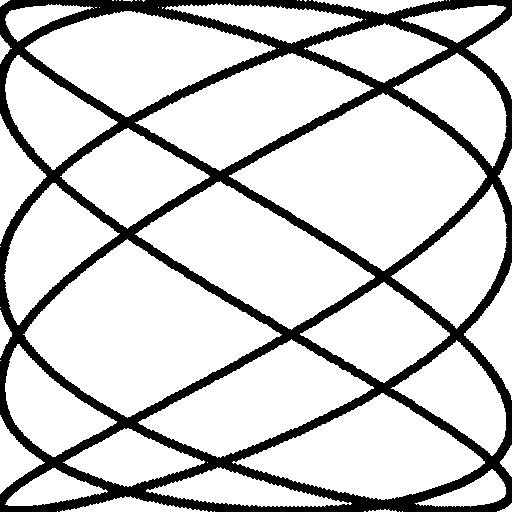Solution: See oscilloscope.py.

8. Bouncing ball with tracks. Modify bouncingball.py to produce images like the ones shown earlier in this page, which show the track of the ball on a gray background.

9. Bouncing ball with gravity. Modify bouncingball.py to incorporate gravity in the vertical direction. Add calls to `stdaudio.playFile()` to add one sound effect when the ball hits a wall and a different one when it hits the floor.

10. Random tunes. Compose a program that uses `stdaudio` to play random tunes. Experiment with keeping in key, assigning high probabilities to whole steps, repetition, and other rules to produce reasonable melodies.

11. Tile patterns. In a previous exercise you wrote programs to create tile-like designs. Using your solution that exercise, compose a program `tilepattern.py` that takes a command-line argument `n` and draws an `n`-by-`n` pattern, using the tile of your choice. Add a second command-line argument that adds a checkerboard option. Add a third command-line argument for color selection. Using the patterns below as a starting point, design a tile floor. Be creative!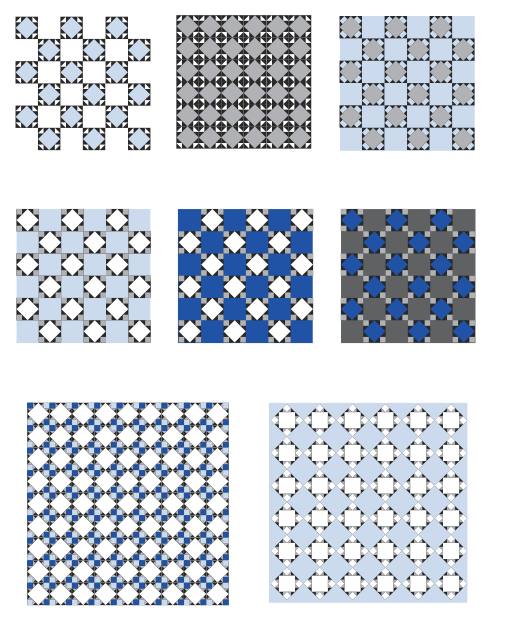Note: These are all designs from antiquity that you can find in many ancient (and modern) buildings such as from from San Giovanni in Laterno (Basilica of St. John Latern) in Rome [ 1 2 3 4 5 6 ] or from the Tile Museum in Lisbon [ 1 2 3 4 5 6 7 8 9 10 ]